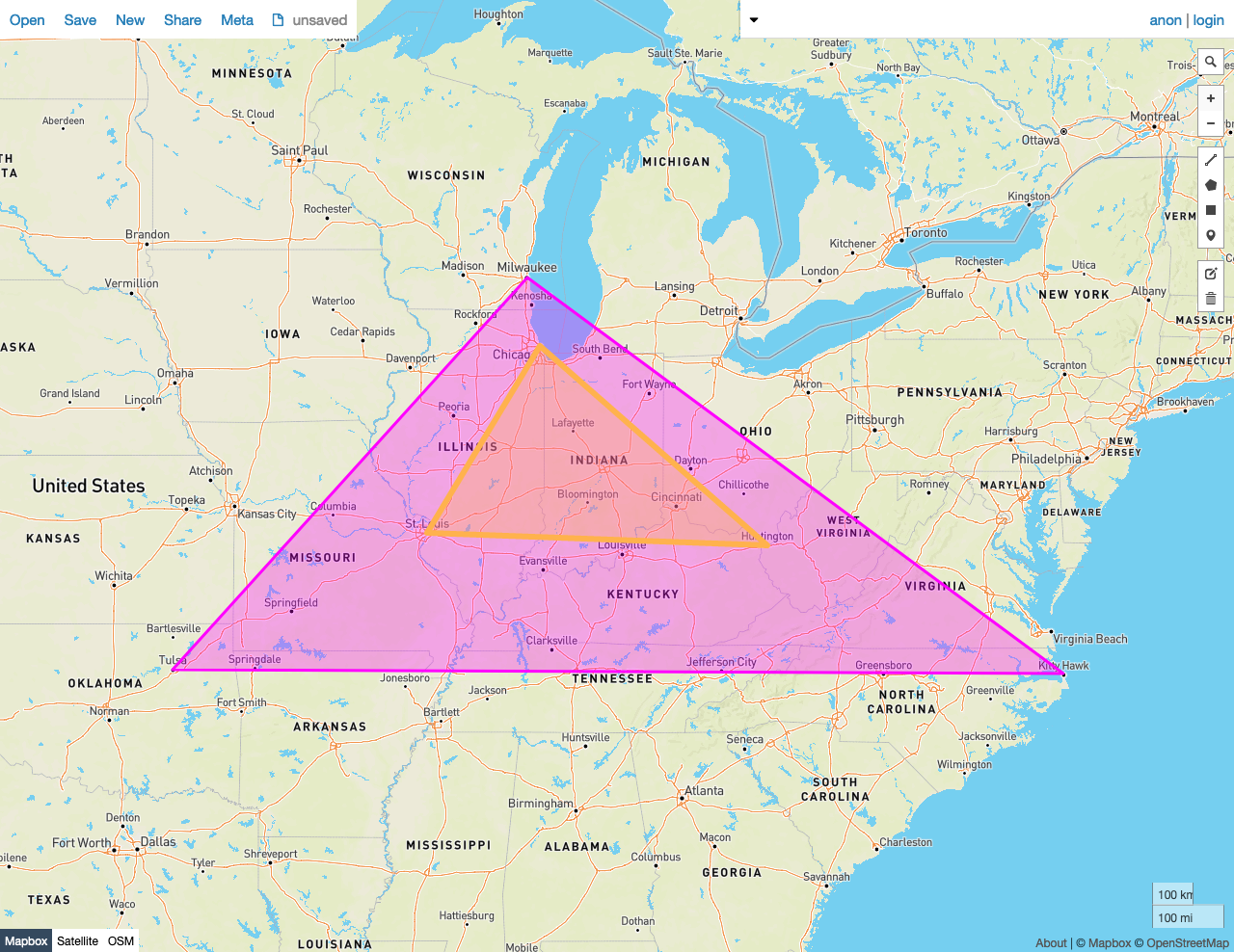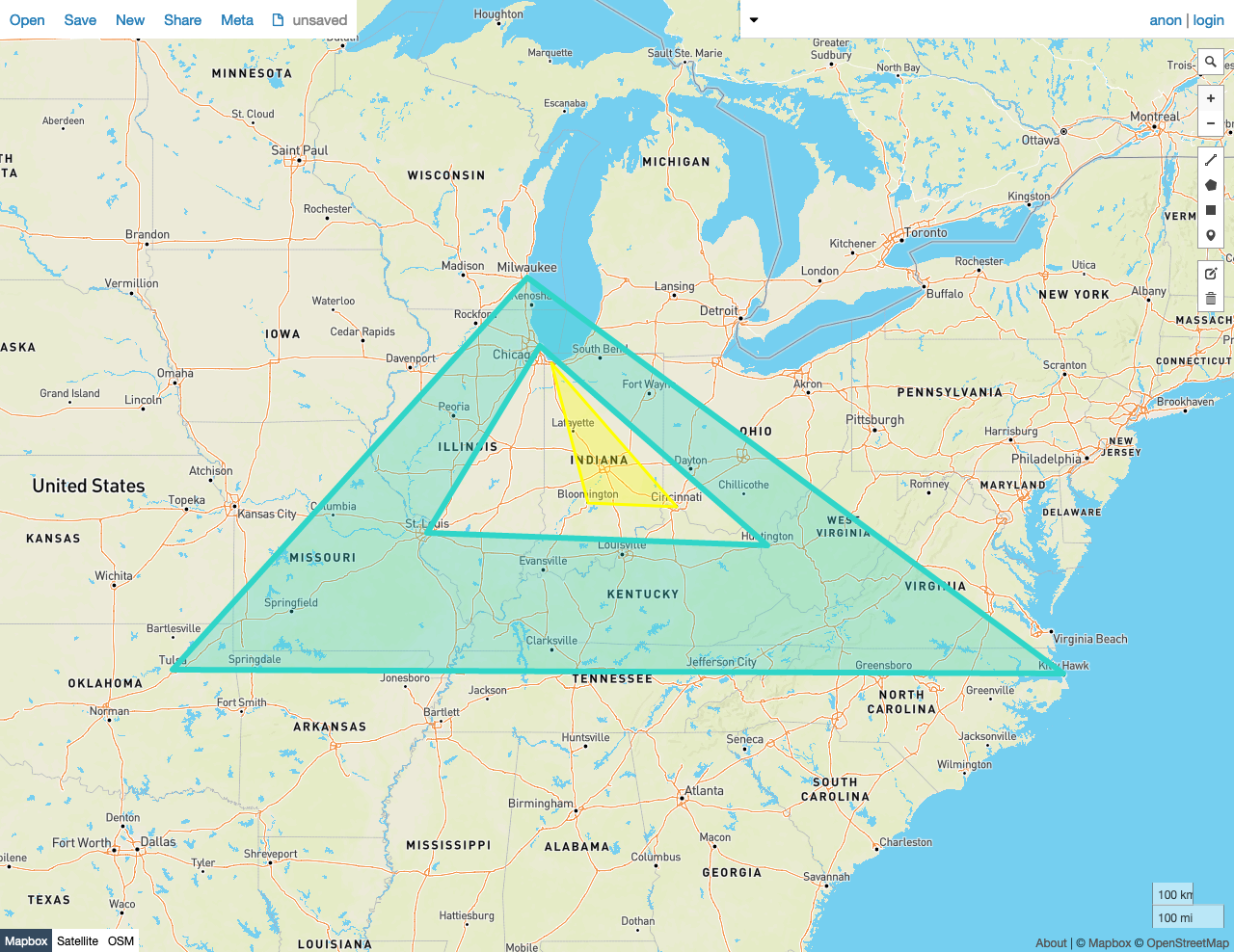Given two shapes A and B, the predicate function `ST_Within(A, B)` returns `true` if the following criteria are met:

• No point in A lies outside of B.
• At least one point in the interior of A lies in the interior of B.

In other words, the exterior of shape B must not include any point in A, and one or more points of A's interior must lie to the interior of B.

This behavior is similar to `ST_CoveredBy`, except that the criteria are more exacting, and therefore some pairs of shapes will be rejected by this function that would be accepted by `ST_CoveredBy`.

`ST_Within` works on the following spatial data types:

Note:

`ST_Within` will attempt to use any available spatial index to speed up its operation. Use the prefixed variant `_ST_Within` if you do not want any spatial indexes to be used.

Note:

This function is the inverse of `ST_Contains`.

## Examples

Note:

The screenshots in these examples were generated using geojson.io, but they are designed to showcase the shapes, not the map. Representing `GEOMETRY` data in GeoJSON can lead to unexpected results if using geometries with SRIDs other than 4326 (as shown below).

### True

In this example, `ST_Within` returns `true` because:

• No point in Polygon A lies outside of Polygon B.
• At least one point in the interior of Polygon A lies in the interior of Polygon B.
``````SELECT ST_Within(st_geomfromtext('SRID=4326;POLYGON((-87.623177 41.881832, -90.199402 38.627003, -82.446732 38.413651, -87.623177 41.881832))'), st_geomfromtext('SRID=4326;POLYGON((-87.906471 43.038902, -95.992775 36.153980, -75.704722 36.076944, -87.906471 43.038902))'));
``````
``````   st_within
---------------
true

(1 row)
``````### False

In this example, `ST_Within` returns `false` because:

• All points in Polygon A lie outside of Polygon B.
``````SELECT ST_Within(st_geomfromtext('SRID=4326;POLYGON((-87.906471 43.038902, -95.992775 36.153980, -75.704722 36.076944, -87.906471 43.038902), (-87.623177 41.881832, -90.199402 38.627003, -82.446732 38.413651, -87.623177 41.881832))'), st_geomfromtext('SRID=4326;POLYGON((-87.356934 41.595161, -84.512016 39.103119, -86.529167 39.162222, -87.356934 41.595161))'));
``````
``````   st_within
---------------
false
(1 row)
``````